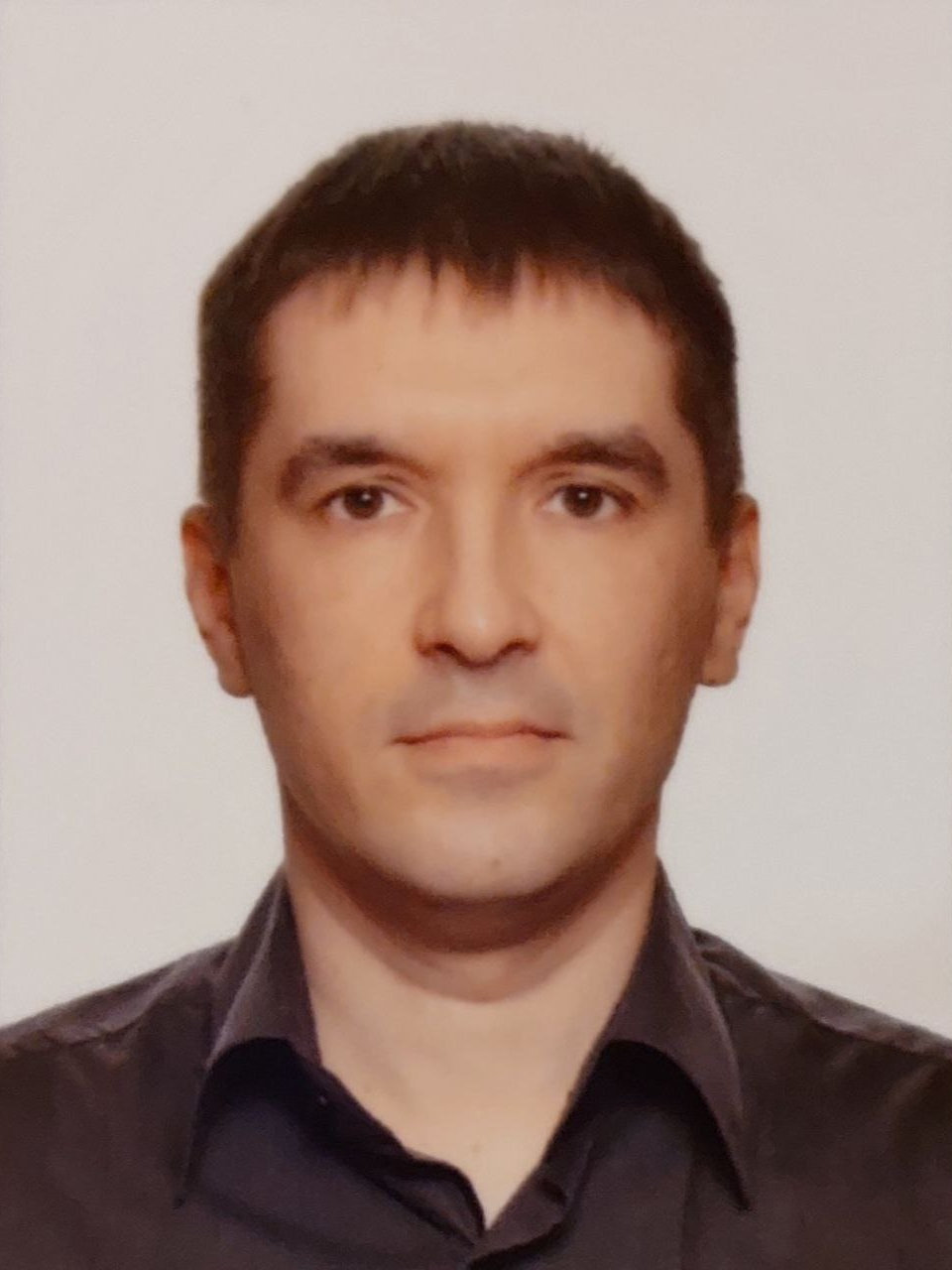Українською | In English

Semen V. Bodnarchuk

Associate professor of the departmentWorks in KPI since 2010

Teaches on faculties: FIOT, FMF

Courses taught:
FICS – Mathematical analysis, Linear algebra and analytic geometry;
FPM – Discrete mathematics, Probability theory and mathematical statistics.

Сторінка викладача в системі Інтелект

Lectures schedule (in Ukrainian) на rozklad.kpi.ua

Education:

Taras Shevchenko National University of Kyiv, 2008, The Faculty of Mechanics and Mathematics, Master of Mathematics

Candidate of sciences in physics and mathematics

Scientific interests:

Stochastic differential equation with Lévy noise

Latest papers:

1) Bodnarchuk S. V., Kulik A. M. Conditions for the existence and smoothness of the distribution density of the Ornstein-Uhlenbeck process with Lévy noise // Theory of Probability and Mathematical Statistics – 2009. – №79. – P. 23–38.

2) Bodnarchuk S. V., Kulik A. M. Conditions of smoothness for the distribution density of a solution of a multidimensional linear stochastic differential equation with Lévy noise // Ukrainian mathematical journal. – 2011. – V. 63, №4. – С. 501–515.